# Texas Go Math Grade 2 Lesson 2.2 Answer Key Compare Numbers

Refer to our Texas Go Math Grade 2 Answer Key Pdf to score good marks in the exams. Test yourself by practicing the problems from Texas Go Math Grade 2 Lesson 2.2 Answer Key Compare Numbers.

## Texas Go Math Grade 2 Lesson 2.2 Answer Key Compare Numbers

Explore

Draw quick pictures to solve the problems. Then complete the sentences.
More ____________ were at the zoo.
Fewer ____________ were at the park.

FOR THE TEACHER: Read the following problem and have children draw quick pictures to compare the numbers. There were 82 butterflies and 86 birds at the zoo. Were there more butterflies or more birds? Repeat for the following problem: There were 47 water fountains at the park and 92 benches at the park. Were there fewer benches or fewer water fountains?
More birds were at the zoo
Fewer water fountains at the park.
Explanation:
The number of butterflies at the zoo=82
The number of birds at the zoo=86
Now comparing the butterflies and birds each other:
When we have two numbers or quantities to compare, then we use three basic symbols, they are:
1. Equal to (=)
2. Greater than symbol (>)
3. less than symbol (<).
Birds are greater than butterflies.
Birds < butterflies.
Therefore, more birds are there in a zoo compared to butterflies.
The number of water fountains in a park=47
The number of benches at the park=92
Now comparing both;
water fountains are lesser than benches.
water fountains<benches.

Math Talk
Mathematical Processes

Explain how you compared the numbers in the first problem.
For comparing numbers there are some basic rules we need to learn:
When we have two numbers or quantities to compare, then we use three basic symbols, they are:
1. Equal to (=)
2. Greater than symbol (>)
3. less than symbol (<).
Here we need to compare the numbers. There are certain rules, based on which it becomes easier to compare numbers. These rules are:
– Numbers with more digits
– Numbers starting with a larger digit
Rule 1: Number with more digits
When we compare numbers, then check if both the numbers are having the same number of digits or not. If a number has more digits, then it is greater than the other number.
For example:
– Two-digit numbers are greater than single-digit numbers
– Three-digit numbers are greater than two-digit numbers and so on…
Rule 2: Numbers starting with a greater digit
This rule is applicable when two numbers are having the same number of digits. In such cases, we need to check the digit at the leftmost place, whichever is greater. Therefore, the number with a greater digit at the leftmost place of the number is greater than the other number.
For example, take the number of birds and butterflies.
birds=86
butterflies=82
86>82 [ 86 is greater than 82]
82<86 [82 is less than 86]
Thus, in the above examples, we can see that, when we compare the two numbers, though the number of digits is the same, one number is greater than/less than the other number.
This is how we differentiate and compare the numbers.

Model and Draw

Use place value to compare numbers. Start by looking at the digits in the greatest place value position first.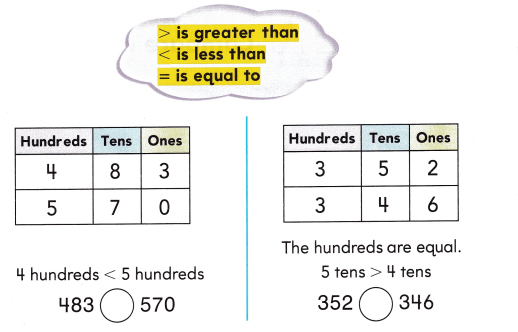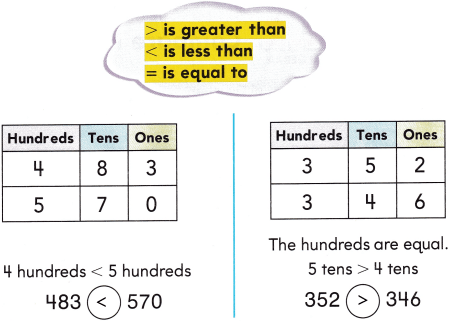Explanation:
Here we need to compare the place values:
In the first diagram,
there are two numbers: 483 and 570
In the number 483,
4 is in the hundreds place
8 is in the tens place
3 is in the one’s place.
In the number 570,
5 is in the hundreds place
7 is in the tens place
0 is in the ones.
Now compare all the places:
First, compare the hundreds of places:
In the hundreds place, 4 and 5 numbers are there:
4 is less than the 5 (4<5)
Now compare tens place :
In the tens place, 8 and 7 are there:
8 is greater than the 7 (8>7)
In the one’s place, 3 and 0 are there:
3 is greater than 0 (3>0).
Likewise, we need to write for 352 and 346.
Compare hundreds, tens and ones place.
3 and 3 are equal (3=3)
5 and 4: 5 is greater than 4 (5>4)
2 and 6: 2 is less than 6 (2>6)

Share and Show

Compare the numbers. Write Is greater than, is less than, or ¡s equal to. Write>, <,or =.

Question 1.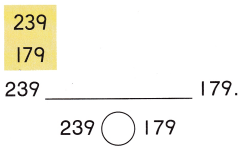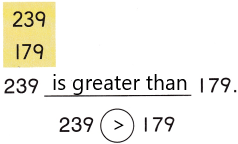Explanation:
By comparing two numbers 239 and 179:
239 is greater than 179.
Fact: In mathematics, the greater than symbol is a basic mathematical symbol that is used to represent the inequality between two values. The symbol used to represent the greater than inequality is “ > “. This is the universally adopted math symbol of two equal length strokes joining in the acute angle at the right.
The typical use of the greater than symbol is to compare two values in which the first number is greater than the second number or one value is greater than the other one. The greater than symbol is an approximation of a closing angle bracket. We can see its huge application in descending order, where the arrangement from the largest number to the smallest number is done using greater than a symbol.

Question 2.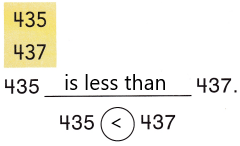Explanation:
By comparing two numbers 435 and 437
435 is less than 437.
Fact: In Mathematics, the less-than symbol is a fundamental Mathematical symbol that describes the inequality between two values. There are different symbols in Maths, which are used to perform different arithmetic operations, hold some constant values, as well as used to compare the quantities with the help of equality and inequality symbols.
The Less-than symbol is to compare two values in which the first number is less than the second number, such as in ascending order. The less than symbol is an approximation of the opening angle bracket. The symbol used to represent the less than inequality is “< “. Less than sign is a universally adopted math symbol of two equal measure strokes that meet in the acute angle at the left.

Question 3.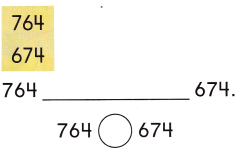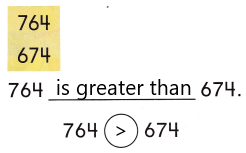Explanation:
By comparing two numbers 764 and 674
Here we apply rule 2:
Rule 2: Numbers starting with a greater digit
This rule is applicable when two numbers are having the same number of digits. In such cases, we need to check the digit at the leftmost place, whichever is greater. Therefore, the number with a greater digit at the leftmost place of the number is greater than the other number.
Applying by above rule now we compare the numbers:
764 and 674: 764 is greater than 674.
The left side digit is greater than the other side. So it is greater.

Question 4.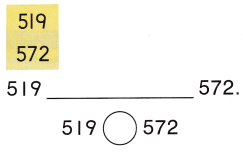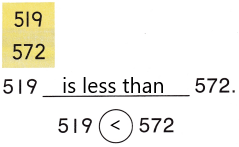Explanation:
– Since, both the numbers, 519 and 572 have three digits, thus we will compare the left-most digit of both the numbers.
5=5, both are equal.
– Now compare the second most digits 1 and 7
1<7
Therefore, 519 < 572
Hence, 519 is less than 572.

Problem Solving

Compare the numbers. Write is greater than, is less than, or is equal to. Write >, <, or =.

Question 5.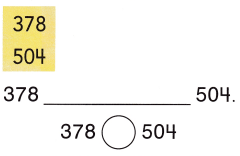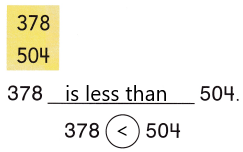Explanation:
– Since, both the numbers, 378 and 504 have three digits, thus we will compare the left-most digit of both the numbers.
– Now compare the second most digits 3 and 5
3<5
Therefore, 378 < 504
Hence, 378 is less than 504.

Question 6.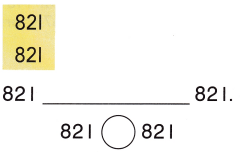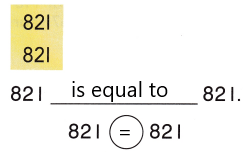Explanation:
– 821 and 821 both the numbers have an equal number of digits, therefore, we will compare the left-most digit of both the numbers.
– As we can see the first three digits are the same for both the numbers, thus we can say both the numbers are equal
Hence, 821 is equal to 821.

Question 7.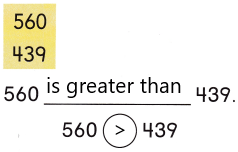Explanation:
Since, both the numbers, 560 and 439 have three digits, thus we will compare the left-most digit of both the numbers.
5 > 4
Therefore,
560 > 439
Hence, 560 is greater than 439.

Question 8.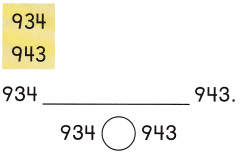Explanation:
– 934 and 943 both the numbers have an equal number of digits, therefore, we will compare the left-most digit of both the numbers.
– But the left-most digit is also the same for both numbers, i.e.,9. So, we need to check the second left-most digit.
– 3 < 4
therefore,
934 < 943
Hence, 934 is less than 943.

Solve. Write or draw to explain.

Question 9.
H.O.T. Mrs. York has 300 red stickers, 50 blue stickers, and 8 green stickers. Mr. Reed has 372 stickers. Who has more stickers?Explanation:
The number of red stickers York has=300
The number of blue stickers York has=50
The number of green stickers York has=8
The total number of stickers York has=X
Add all the numbers of red, blue, green stickers.
x=300+50+8
X=358.
Therefore, Mrs York has 358 stickers.
The number of stickers Reed has=372
Now compare both the numbers 358 and 372
358 and 372  both the numbers have an equal number of digits, therefore, we will compare the left-most digit of both the numbers.
– But the left-most digit is also the same for both numbers, i.e.,3. So, we need to check the second left-most digit.
5 < 7
Therefore,
358 < 372
Hence, 358 is smaller than 372.

Question 10.
H. O.T. Multi-Step Use the digits on these cards to make two 3-digit numbers. Use each card only once. Compare the numbers.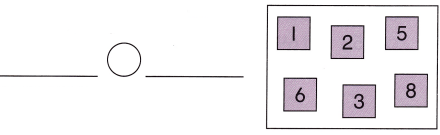Explanation:
Since, both the numbers, 125 and 638 have three digits, thus we will compare the left-most digit of both the numbers.
1 < 6
Therefore,
125 < 638
Hence, 125 is less than 638.

Question 11.
Analyze A spruce tree has lived for 584 years. A fir tree has lived for 198 years. A redwood tree has lived for 767 years. Which is the least number of years?
(A) 584
(B) 767
(C) 198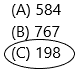Explanation:
The number of years a spruce tree has lived=584
The number of years a fir tree has lived=198
The number of trees a redwood tree has lived=767
We need to find out the least number of years a tree has lived.
compare the numbers 1 is the least number so 198 is the least.

Question 12.
Analyze Mario sees the numbers 701, 149, and 589. Which number has the greatest number of hundreds?
(A) 701
(B) 149
(C) 589Explanation:
The numbers Mario analyzes 701, 149, 589
We need to find out the greatest number:
So that check the place values of hundreds place.
The hundreds place the numbers are 7, 1, and 5
7 is the highest number.
Hence, 701 is greater than other two numbers.

Question 13.
Isaiah has 399 stickers in one book, 600 stickers in another book, and 599 stickers in a third book. Which is the greatest number?
(A) 399
(B) 600
(C) 599Explanation:
The number of stickers in the first book=399
The number of stickers in the second book=600
The number of stickers in the third book=599
We need to find out the greatest number
Check the place values first:
In the hundreds place, the numbers are 3, 6, and 5
600 is the highest
Hence, 600 is greater than the other two numbers.

Question 14.
TEXAS Test Prep Which of these is true?
(A) 453 > 354
(B) 253 < 164
(C) 391 > 417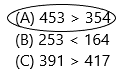Explanation:
453 > 354 both the numbers have an equal number of digits, therefore, we will compare the left-most digit of both numbers.
4 > 3
Therefore,
453 > 354
hence, 453 is greater than 354.

TAKE HOME ACTIVITY • Have your child explain how to compare the numbers 281 and 157.